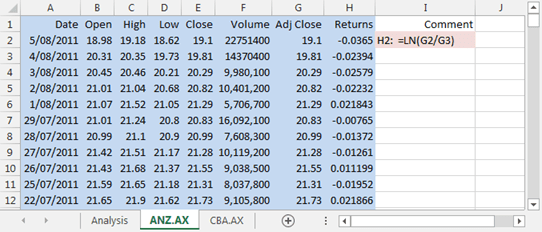# Vlookup w/ indirect

## Source data

An analyst has a series of worksheets containing sample price and volume data for several stocks traded on the Australian Stock Exchange (ASX). The stock data for ANZ bank is shown in figure 1.

The download data from Yahoo Finance includes the series labels Date to Adj Close as shown in columns A to G of the ANZ.AX worksheet. The analyst has added the log return column, labeled Returns in column H with the Excel LN function used to create the vector $$r_t = log(P_{t} / P_{t-1})$$Fig 1: Price and volume data for ANZ bank. The range shown in columns A to H, coloured blue is named ANZ, with worksheet ANZ.AX scope.

A summary worksheet named Analysis has been created, and the analyst wants to use a master list of trading days to collate the return data for stock codes ANZ and CBA. The summary sheet is shown in figure 2.Fig 2: The summary worksheet named Analysis. A master list of trading days is a column vector that starts at cell A14.

The best way to collate the individual return series is by using the VLOOKUP function with an exact match based on dates in the Date vector. This means, if the date from the master list cannot be found in the table_array, then VLOOKUP will return a #N/A error. The analyst should never simply copy and paste from the source worksheets as there is no certainty of one to one matching.

There are several ways to write the lookup formula. These are discussed next.

## Writing the lookup function

• SYNTAX: INDIRECT(ref_text,a1)
• SYNTAX: VLOOKUP(lookup_value,table_array,col_index_num,range_lookup)

### VLOOKUP w/ direct reference

• Refer to the Analysis1 worksheet in the Excel Web App window below.
• The formula in cell B14:  =VLOOKUP($A14,ANZ.AX!ANZ,8,FALSE) • The lookup_value is the date that is always in column A of the sample, and is in the same relative row of the formula.$A indicates absolute column A, and 14, without the $prefix is the same row as the formula. • The source data is a range named ANZ with worksheet scope on the ANZ.AX worksheet. Thus the table_array must include the worksheet name, indicated by the semicolon, and the worksheet scope range name. The entry as a worksheet and range combination is ANZ.AX!ANZ. • The VLOOKUP function looks for the date in column 1 of table_array, and returns the corresponding value from column 8, as indicated by the col_index_num of 8. • If the exact date cannot be found, then VLOOKUP will return #N/A. • The formula in cell B14 can be copied down to the other cells in column B, but will need to be manually updated to CBA.AX!CBA in column C. ### VLOOKUP w/ INDIRECT reference • Refer to the Analysis2 worksheet in the Excel Web App window below. • In this case the formula is – B14:  =VLOOKUP($A14,INDIRECT(B$13&".AX!"&B$13),8,FALSE)
• In this example, the stock code is always in row 13, and in the same relative column as the formula. Absolute row 13 is written as $13. • When B13: ANZ, then B$13&”.AX!”&B\$13 returns “ANZ”&”.AX!”&”ANZ” with value ANZ.AX!ANZ. This is simply a text string, thus the INDIRECT function is used to point to the ANZ.AX!ANZ range.
• This style of formula is easily replicated both vertically and horizontally, and is a common solution to this particular task.

Fig 1: Excel Web App #1: Analysis and Data worksheets. VLOOKUP function w/ INDIRECT on Analysis2.

• This example was developed in Excel 2013 Pro 64 bit.